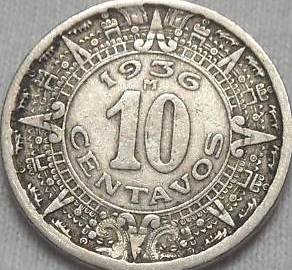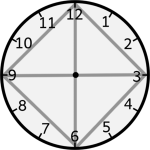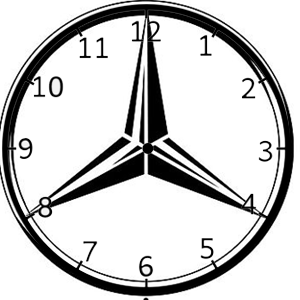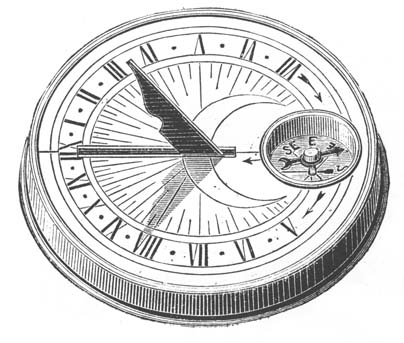Circle geometry II: 3, 6, 9

Twelve spokes, one wheel, navels three.
Who can comprehend this?
On it are placed together
three hundred and sixty like pegs.
They shake not in the least.

Dirghatamas, Rigveda 1.164.48 c. 1500–1200 B.C.I’m not clever in math. I can notice some basic relationships, though, and I can see that both the 12 hour clock and the 360° circle are divisible by 6’s (and of course, 3’s). On the clock face, the numbers 3, 6, 9, and 12 can be connected with lines to form diamond with a plus sign (+). The plus sign is also a visual representation of four 90° angles – the 360° of a circle or the four interior angles of a square.Like the numbers on the clock and the degrees in a circle, the Persian calendar 360 day year is divisible by 6’s and 3’s and suggests that the degrees on a circle may be partly a graphical representation of a year. Similarly, our modern calendar is divided, like a clock, into twelve units (months).Aztec and Mayan calendars also take the form of a circle, and design elements from some of these ancient calendars have been used in modern currency.

Threes and ThirdsIf there are 360° in a circle, then 1/3 of the circle is 120°. 120° x 3 = 360. Half of a circle is 180°, same as the interior angles of a triangle (60° x 3). Two circles would be 360° x 2 or 720°, the same as the interior angles of a hexagon (120° x 6).

The Mercedes-Benz Logo features a circle divided into thirds. That means that each interior angle would be 120°. If it were a clock, its “hands” would be pointing to 12:00, 4:00, 8:00.12, the number of the Hanged Man, represents the last month in the year, the top number on a wall clock, and the middle point (midday) on a Sun Dial.3 x 3 = 9. The fraction 1/3 is represented by an infinitely repeating decimal: .33333333 (etc). Another repeating decimal, .999… is said to be equivalent the whole number 1.